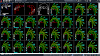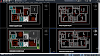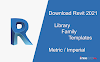### Recents in Beach

ACCESS FREE ENTIRE CAD LIBRARY DWG FILES Download free AutoCAD drawings of architecture, Interiors designs, Landscaping, Constructions detail, Civil engineer drawings and detail, House plan, Buildings plan, Cad blocks, 3d Blocks, and sections.
.

Builder Calculator - Concrete Volume Calculator is a simple tool to calculate the volume of footings, slab, square column, round column, and steel weight. It also calculates the total cost and quantity of concrete needed for the construction of footings, slab, square column, and round column.

Builder Calculator - Concrete Volume Calculator features :
- Calculates volume and total cost for different types of footings, slab, square column, and round column.
- Calculates weight and total cost for different types of steel.
- Calculates concrete mix – Amount of concrete cement, sand, gravel, and water for a given volume.
- How many premix concrete bags required for your project.
-Edit the option to set your own bag size and rate of premix bags.
- Calculates the quantity of bricks needed to build a wall.
- Calculates the quantity of bricks needed to build a circular wall.
- Calculates the quantity of blocks needed to build a wall.
- Unit converters ( length unit converter, area unit converter, volume unit converter, and weight unit converter ).
- Metric or imperial Units support
- It is free, fast, simple, and easy to use.

Article source
http://www.civilstudent.com/